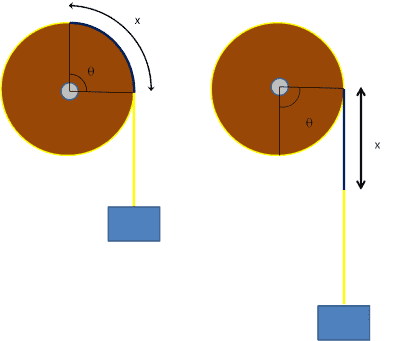# Inertia of a flywheel, with a mass attached in a cord.

## Homework Statement

Hi :)

So we've been given an exercize, where we have to calculate the inertia of a rotating flywheel in three different methods. The setup can been seen below.First by measuring and calculating the diameter, mass, radius, volume etc. Worked out just fine.

The second is causing the troubles. Measuring and calculating it by using the laws of conservation of energy.

The third was using it as pendule. Worked out fine aswell, with a good result, very close to the first method.

## Homework Equations

So we've been trying to solve it, by the means of laws of conservation, where we first neglect the friction.

Loss in potential energy = increase in kinetic energy.

So by using the above statement, we can calculate the inertia. The wheel is not moving along a horisontal axis, so therefor there is no velocity:

$mgh = \stackrel{1}{2} I \omega^2$ Solving for I

$I = \stackrel{2mgh}{\omega^2}$

Which gave a okay nice solution.

## The Attempt at a Solution

Then we now consider the friction as a force acting against the wheel. By making two equations, using Newtons Second Law of Rotation (τ = Iα), from t0 to t1 and from t1 to t2.

t0 - t1 is where the mass is still attached to the wheel. From t1 - t2, the mass has run out of cord and is no longer affecting the wheel with a downward force. In other words, the cord has slipped the wheel. So here's the two equations:

First from t0-t1

$I α = tc r$ (tc is the force from the cord/tension)$→ tc = \stackrel{Iα}{r}.$

Using Newtons Second law, and by writing a free body diagram, following equation can be written:

$ma = tc - mg → ma = \stackrel{Iα}{r} -mg$

Rewriting a to α and solving for I.

$mαr = \stackrel{Iα}{r} -mg → mαr^2 = Iα -mgr → mαr^2 + mgr = Iα → I =mr^2+\stackrel{mgr}{α}$

Substituting this expression in the general torque equation and putting the friction T, as a force in we get:

$Iα = (mr^2+\stackrel{mgr}{α})α - T$ [t0,t1]

And since the mass is 0 from [t1,t2]:

$Iα = -T$

And substituting the mass, angular acceleration, gravity and radius, and solve for I and T, we get a completely other answer, way off method 1 and 3.

I for Method 1 ≈ 0,31

I for Method 2 ≈ 4,2

I for Method 3 ≈ 0,19

So my question is, what am I doing wrong? For me the theory for method 2 seems correct, but there must be an error somewhere :)